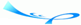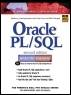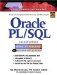# Chapter 8 Iterative Control

 Team-FlyOracle® PL/SQL® Interactive Workbook, Second EditionBy Benjamin Rosenzweig, Elena Silvestrova Table of Contents Appendix D.  Answers to Test Your Thinking Sections

 1) Rewrite script ch08_1a.sql using a WHILE loop instead of a simple loop. Make sure that the output produced by this script does not differ from the output produced by the script ch08_1a.sql. A1: Answer: Consider the script ch08_1a.sql:` SET SERVEROUTPUT ON DECLARE v_counter BINARY_INTEGER := 0; BEGIN LOOP -- increment loop counter by one v_counter := v_counter + 1; DBMS_OUTPUT.PUT_LINE ('v_counter = '||v_counter); -- if EXIT condition yields TRUE exit the loop IF v_counter = 5 THEN EXIT; END IF; END LOOP; -- control resumes here DBMS_OUTPUT.PUT_LINE ('Done…'); END; `Next, consider a new version of the script that uses a WHILE loop. All changes are shown in bold letters.` SET SERVEROUTPUT ON DECLARE v_counter BINARY_INTEGER := 0; BEGIN WHILE v_counter < 5 LOOP -- increment loop counter by one v_counter := v_counter + 1; DBMS_OUTPUT.PUT_LINE ('v_counter = '||v_counter); END LOOP; -- control resumes here DBMS_OUTPUT.PUT_LINE('Done…'); END; `In this version of the script, you replace a simple loop by a WHILE loop. It is important to remember that a simple loop executes at least once because the EXIT condition is placed in the body of the loop. On the other hand, a WHILE loop may not execute at all because a condition is tested outside the body of the loop. So, in order to achieve the same results using the WHILE loop, the EXIT condition` v_counter = 5 `used in the original version is replaced by the test condition` v_counter < 5 `When run, this example produces the following output:` v_counter = 1 v_counter = 2 v_counter = 3 v_counter = 4 v_counter = 5 Done… PL/SQL procedure successfully completed. ` 2) Rewrite script ch08_4a.sql using a simple loop instead of a numeric FOR loop. Make sure that the output produced by this script does not differ from the output produced by the script ch08_4a.sql. A2: Answer: Recall the script ch08_4a.sql:` SET SERVEROUTPUT ON DECLARE v_factorial NUMBER := 1; BEGIN FOR v_counter IN 1..10 LOOP v_factorial := v_factorial * v_counter; END LOOP; -- control resumes here DBMS_OUTPUT.PUT_LINE ('Factorial of ten is: '|| v_factorial); END; `Next, consider a new version of the script that uses a simple loop. All changes are shown in bold letters.` SET SERVEROUTPUT ON DECLARE v_counter NUMBER := 1; v_factorial NUMBER := 1; BEGIN LOOP v_factorial := v_factorial * v_counter; v_counter := v_counter + 1; EXIT WHEN v_counter = 10; END LOOP; -- control resumes here DBMS_OUTPUT.PUT_LINE ('Factorial of ten is: '|| v_factorial); END; `In this version of the script, you replace a numeric FOR loop with a simple loop. As a result, there are three important changes that you should make. First, you need to declare and initialize the loop counter, v_counter. This counter is implicitly defined and initialized by the FOR loop. Second, you need to increment the value of the loop counter. This is very important because if you forget to include the statement` v_counter := v_counter + 1; `in the body of the simple loop, you will end up with an infinite loop. The step is not necessary when using numeric FOR loop because it is done by the loop itself.Third, you need to specify the EXIT condition for the simple loop. Because you are computing a factorial of 10, the following EXIT condition is specified:` EXIT WHEN v_counter = 10; `Notice that you could specify this EXIT condition using IF-THEN statement as well:` IF v_counter = 10 THEN EXIT; END IF; `When run, this example shows the following output:` Factorial of ten is: 362880 PL/SQL procedure successfully completed. ` 3) Rewrite script ch08_6a.sql. A simple loop should be used as the outer loop, and a WHILE loop should be used as the inner loop. A3: Answer: Consider the script ch08_6a.sql:` SET SERVEROUTPUT ON DECLARE v_test NUMBER := 0; BEGIN <> FOR i IN 1..3 LOOP D BMS_OUTPUT.PUT_LINE ('Outer Loop'); DBMS_OUTPUT.PUT_LINE ('i = '||i); DBMS_OUTPUT.PUT_LINE ('v_test = '||v_test); v_test := v_test + 1; <> FOR j IN 1..2 LOOP DBMS_OUTPUT.PUT_LINE ('Inner Loop'); DBMS_OUTPUT.PUT_LINE ('j = '||j); DBMS_OUTPUT.PUT_LINE ('i = '||i); DBMS_OUTPUT.PUT_LINE ('v_test = '||v_test); END LOOP inner_loop; END LOOP outer_loop; END; `Next, consider a modified version of the script that uses simple and WHILE loops. All changes are shown in bold letters.` SET SERVEROUTPUT ON DECLARE i INTEGER := 1; j INTEGER := 1; v_test NUMBER := 0; BEGIN <> LOOP DBMS_OUTPUT.PUT_LINE ('Outer Loop'); DBMS_OUTPUT.PUT_LINE ('i = '||i); DBMS_OUTPUT.PUT_LINE ('v_test = '||v_test); v_test := v_test + 1; -- reset inner loop counter j := 1; <> WHILE j <= 2 LOOP DBMS_OUTPUT.PUT_LINE ('Inner Loop'); DBMS_OUTPUT.PUT_LINE ('j = '||j); DBMS_OUTPUT.PUT_LINE ('i = '||i); DBMS_OUTPUT.PUT_LINE ('v_test = '||v_test); j := j + 1; END LOOP inner_loop; i := i + 1; -- EXIT condition of the outer loop EXIT WHEN i > 3; END LOOP outer_loop; END; `Just like in the previous exercise, there are some changes that are important due to the nature of the loops that are used.First, both counters, for outer and inner loops, must be declared and initialized. Moreover, the counter for the inner loop must be initialized to 1 prior to the execution of the inner loop, and not in the declaration section of this script. In other words, the inner loop executes three times. It is important not to confuse the term execution of the loop with the term iteration. Each execution of the WHILE loop causes the statements inside this loop to iterate twice. Before each execution, the loop counter j must reset to 1 again. This step is necessary because the WHILE loop does not initialize its counter implicitly like numeric FOR loop. As a result, after the first execution of the WHILE loop is complete, the value of counter j is equal to 3. If this value is not reset to 1 again, the loop will not execute second time.Second, both loop counters must be incremented. Third, the EXIT condition must be specified for the outer loop, and the test condition must be specified for the inner loop.When run, the exercise produces the following output:` Outer Loop i = 1 v_test = 0 Inner Loop j = 1 i = 1 v_test = 1 Inner Loop j = 2 i = 1 v_test = 1 Outer Loop i = 2 v_test = 1 Inner Loop j = 1 i = 2 v_test = 2 Inner Loop j = 2 i = 2 v_test = 2 Outer Loop i = 3 v_test = 2 Inner Loop j = 1 i = 3 v_test = 3 Inner Loop j = 2 i = 3 v_test = 3 PL/SQL procedure successfully completed. `

 Team-FlyTopOracle PL/SQL Interactive Workbook (2nd Edition)
ISBN: 0130473200
EAN: 2147483647
Year: 2002
Pages: 146